#Interactive Real Analysis

Next | Previous | Glossary | Map

## Ratio Test

### Definition: Euler Series

The seriesis called Euler's series. It converges to Euler's number e.

### Proof:

It is easy to show that this series converges. We could use, for example, the ratio test: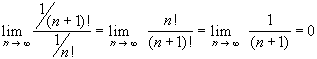Hence, the series converges by the ratio test. However, this test says nothing about the actual limit of the series. We have to be a lot more sophisticated to find the actual limit of this series.

Recall that we have defined Euler's number as the limit of the Euler sequence. The proof that the above sum equals that limit is very similar to the proof that the Euler sequence converges in the first place.

First, we use the binomial theorem to expand the expression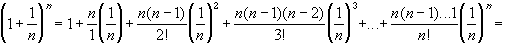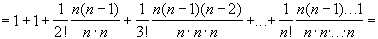From that expansion it is clear that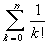because each term in parenthesis is smaller than one. On the other hand, we also have: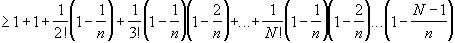for N < n, because each term in parenthesis is greater than zero. But then, taking the limit as n approaches infinity, we have:Hence, taking the limit as N approaches infinity, we have the sum squeezed in between the limit of Euler's sequence, which we know is equal to e. That proves the result.Next | Previous | Glossary | Map